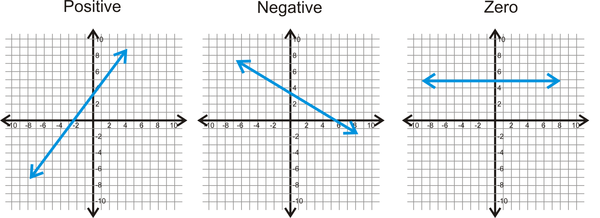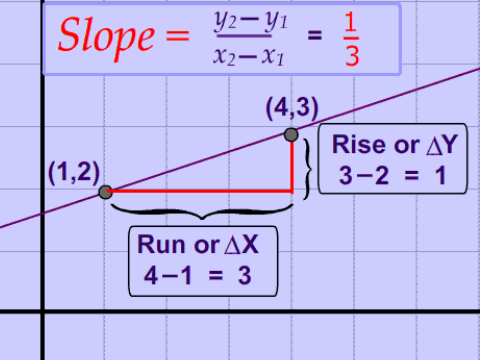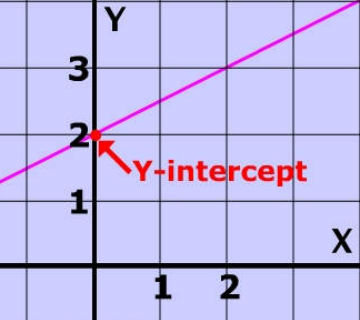Glossary (auto-filter)

Browse the glossary using this index

Special | A | B | C | D | E | F | G | H | I | J | K | L | M | N | O | P | Q | R | S | T | U | V | W | X | Y | Z | ALL

is rise over run.

Learn how to calculate the slope of the line in a graph by finding the change in y and the change in x.Y

y-intercept

of a Straight Line. Where a line crosses the y-axis of a graph. Just find the value of y when x equals 0.